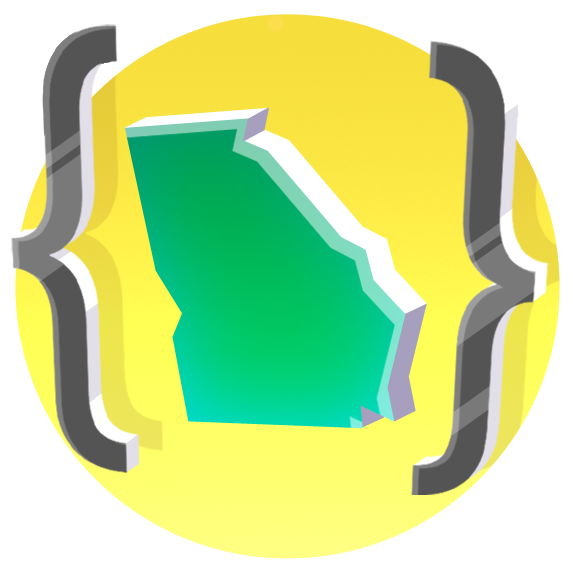### Please enable JavaScript to use CodeHSGeorgia Foundations of Computer Programming

#### DemosTracy will draw a slinky with 5 loops. <hr> As you explore this example, test out the following: * Look at each command. What do you notice about the syntax, or the way each command is written? * Change the order of the commands. How does the functionality of the program change? * Make the following changes and note their effect including any error messages produced: * Capitalize the first letter in one of the `circle` commands * Remove the parentheses in the first command * Remove the closing parenthesis in the first command * Add a blank line between the commands on the 2nd and 3rd lines * Indent only the first command * Indent only the second command * What happens if every numerical value in the program (inside all `circle` and `forward` commands) is the same number? What do you notice about the spacing between circles? * How must the numbers in the `circle` and `forward` commands be altered to have the circles line up next to one another instead of overlapping?### Circle Square Triangle

This code will draw a red circle, blue square, and yellow triangle all using the `circle()` command. <hr> As you explore this example, test out the following: * What happens if you draw the yellow triangle first and the red circle last? * What happens if you use the advanced circle command with 10 steps to draw the red circle (`circle(75, 360, 10)`)? * Do some testing. How many steps do you feel are required for a shape to look like a circle? * Change the radius of the circle to 150. (*Note: The shape will extend off of the canvas.*) Does this same number of steps still result in a shape that looks like a circle?